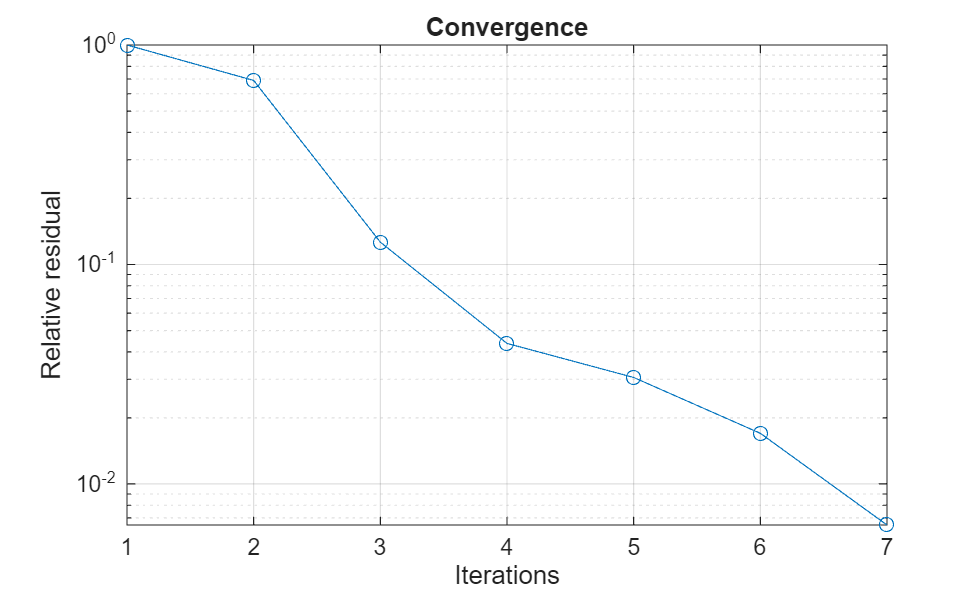# Analysis of Electrically Large Structures Using Hybrid MoM and FMM

This example shows how to analyze electrically large antennas using two solvers in Antenna Toolbox™: the hybrid method of moments (MOM) and the fast multipole method (FMM). The overall size of an antenna can be expressed in electrical terms, as a function of the frequency of operation or wavelength $\lambda$. Doing so can enable you to choose the electromagnetic solver to deploy for analysis.

Typical antennas such dipole antennas and microstrip patch antennas have a radiating element size of $\frac{\lambda }{2}$. Including a ground plane can increase the overall dimensions up to 3$\lambda$. However, certain classes of antennas, by their intended application are electrically large. A common example of such antennas is the parabolic reflector. These antennas have an electrical size in the range of 5$\lambda$ to 100$\lambda$.

To solve these structures, you must discretize the geometry. In Antenna Toolbox, metal surfaces are discretized into a mesh consisting of triangles. The immediate effect of a larger electrical size is an increase in the size of the mesh results in more unknowns to solve for, during analysis by the solver.

You can use one of two approaches to solving electrically large structures: the first approach is hybrid MoM, which is an approximate technique that relies on the physical optics (PO) method to couple an electrically large portion of the geometry being analyzed to the relatively smaller radiating element being handled by a full-wave MoM technique.

The second approach uses an iterative FMM algorithm to avoid building the interaction matrix, thereby avoiding the storage constraints of a typical direct solver (relying on the explicit matrix inverse), but still produces a solution with full-wave accuracy.

The hybrid MoM approach will trade-off full-wave accuracy in order to be faster whereas the FMM based solver removes the matrix storage requirements of a direct solver thus enabling solution of a electrically large structures with full-wave accuracy.

### Define Reflector Specifications

Define the frequency and physical dimensions of the parabolic reflector. The reflector consists of a $\frac{\lambda }{2}$ dipole as the exciter. The electrical size of this parabolic reflector is $\approx 20\lambda$.

```Fc = 3.2975e9; lambda = physconst('lightspeed')/Fc; D = 2; F_over_D = 0.51;```

### Create Parabolic Reflector

Use the design object function to design a parabolic reflector at the desired center frequency and adjust the properties to align with the physical specifications.

```p = design(reflectorParabolic,Fc); p.Radius = D/2; p.FocalLength = D*F_over_D```
```p = reflectorParabolic with properties: Exciter: [1×1 dipole] Radius: 1 FocalLength: 1.0200 FeedOffset: [0 0 0] Tilt: 0 TiltAxis: [1 0 0] Load: [1×1 lumpedElement] SolverType: 'MoM-PO' ```

Visualize the structure using the `show` object function. Notice that one of the properties in the object display is `SolverType`. By default, the hybrid solver is the solver for antennas of the reflector family.

```figure show(p)```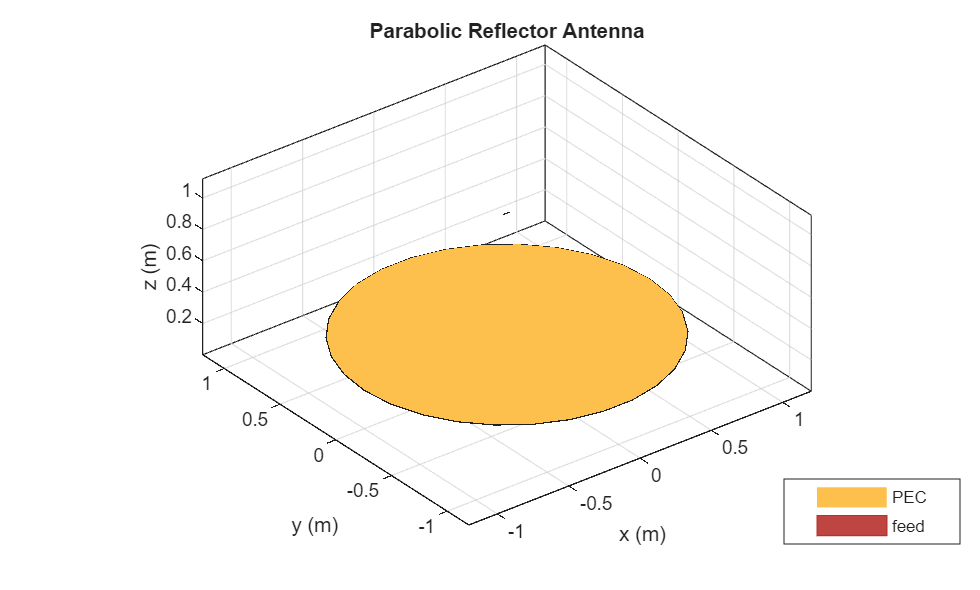Set up a far-field analysis by using the `pattern` function. The default angular spacing for this function in both the azimuth and elevation planes is 5 degrees. Since the antenna is an electrically large reflector antenna, expect a narrow main beam towards the zenith. For this example, specify a finer angular discretization of 1 degrees. in both planes. Use the `PatternPlotOptions` class to adjust the magnitude limits on the overall plot output.

```az = -180:2:180; el = -90:1:90; P = PatternPlotOptions; P.MagnitudeScale = [-10 32]; figure pattern(p,Fc,az,el,patternOptions=P)```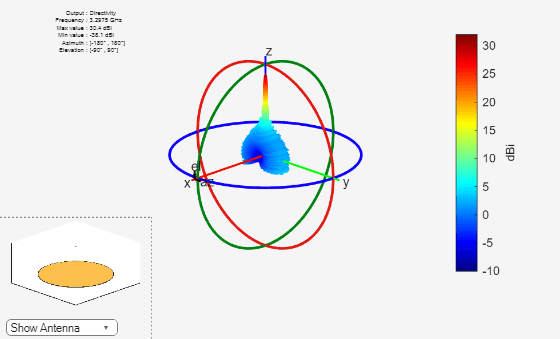Fix the azimuth angle to 90 degrees and change the coordinate system to rectangular to generate a pattern plot that varies with the elevation plane.

```figure pattern(p,Fc,90,el,CoordinateSystem='rectangular');```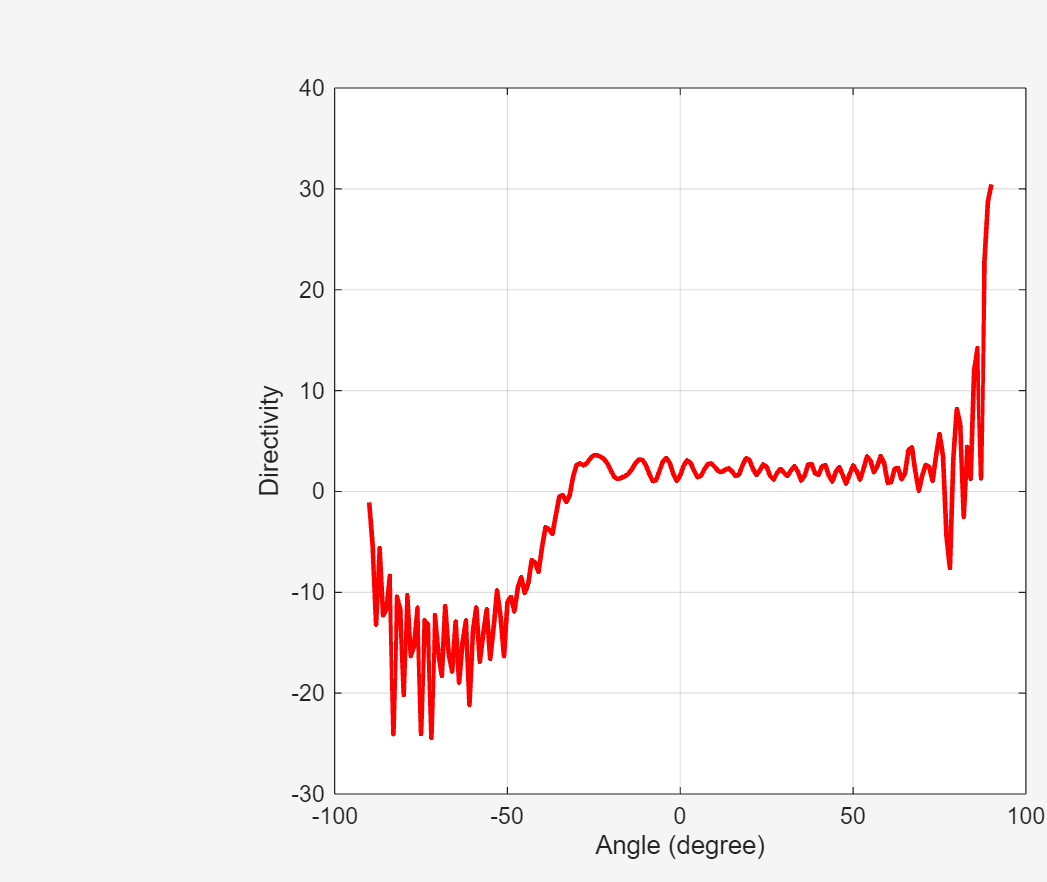### Switch to FMM Solver

Use the `SolverType` property on the reflector and switch it to FMM. The FMM uses an iterative solver to arrive at the final solution. Invoking the object function, `solver`, on the antenna object gives you access to the properties. One of the key advantages with a hybrid solver like MoM-PO is that you can relax the meshing criterion on the structure.

```p.SolverType = 'FMM'; s = solver(p)```
```s = EFIE with properties: Iterations: 100 RelativeResidual: 1.0000e-04 Precision: 2.0000e-04 ```

Because the FMM is a full-wave solver, you must refine the mesh. To understand this, change the default number of iterations to 20 and calculate and visualize the pattern as before.

```s.Iterations = 20; figure pattern(p,Fc,az,el,patternOptions=P)```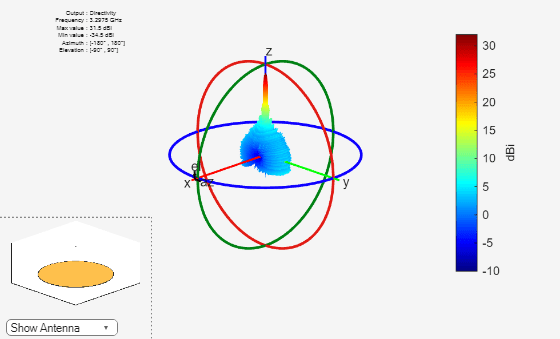### Plot Solver Convergence

The far-field pattern appears similar to that computed from the hybrid MoM method. Plot the convergence of the solver as a function of the number of iterations. The requested `RelativeResidual` in the solver properties is set to `1e-4`, whereas the convergence plot reveals that past the fifth iteration, the convergence slows down dramatically and eventually fails to achieve the desired residual.

The iterative solver solves the system of equations where the V is known and the Z, the interaction matrix, is never formed and stored. The left-hand side is instead computed as a matrix-vector product using the estimated I for each iteration by the GMRES algorithm. The convergence trend observed in the plot suggests that the mesh might need to be refined.

```figure convergence(s)```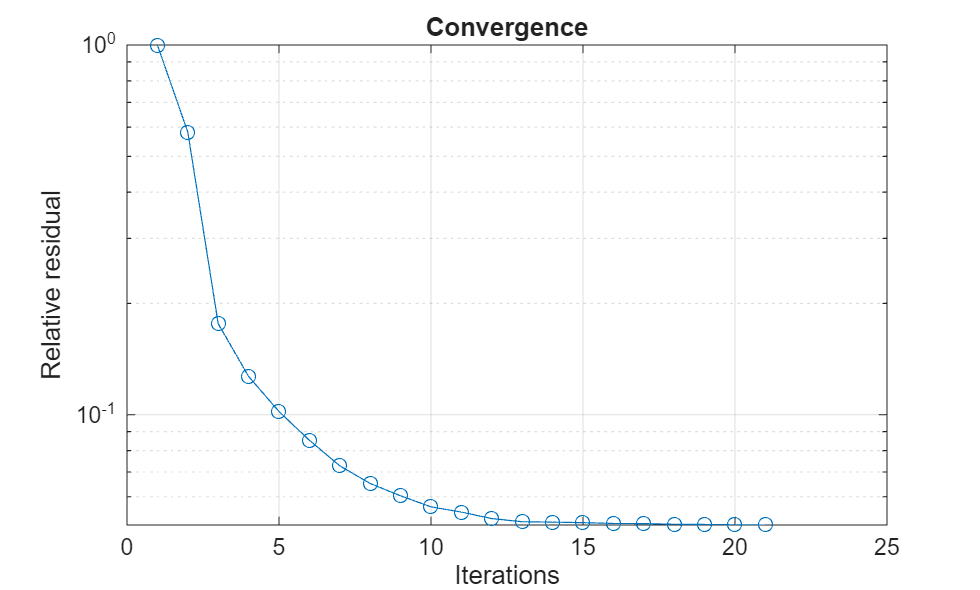### Improve Mesh and Solve

Before refining the mesh, check the ratio of the wavelength to the maximum edge length.

`M = mesh(p)`
```M = MeshReader with properties: Points: [3×2414 double] Triangles: [4×4650 double] Tetrahedra: [] MaxEdgeLength: 0.0391 MinEdgeLength: 0.0293 GrowthRate: 0.7500 MinimumMeshQuality: 0.6548 MeshMode: 'auto' ```
`mesh_ratio = lambda/M.MaxEdgeLength`
```mesh_ratio = 2.3265 ```

Use the `mesh` function to refine the mesh and then recompute the pattern. The solver properties are retained.

`M = mesh(p,MaxEdgeLength=lambda/6)`
```M = MeshReader with properties: Points: [3×16756 double] Triangles: [4×33070 double] Tetrahedra: [] MaxEdgeLength: 0.0152 MinEdgeLength: 0.0114 GrowthRate: 0.7500 MinimumMeshQuality: 0.7497 MeshMode: 'manual' ```

Plot the 3-D pattern and a slice at the azimuth of 90 degrees to show the variation along the elevation plane.

```figure pattern(p,Fc,az,el,patternOptions=P)```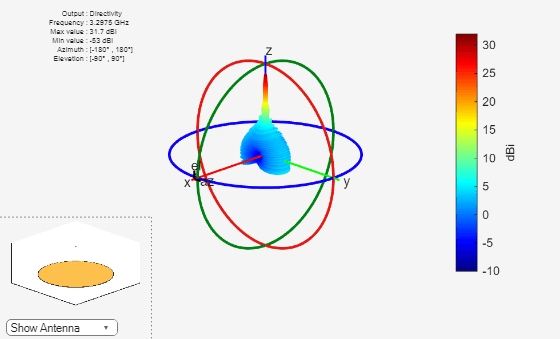```figure pattern(p,Fc,90,el,CoordinateSystem='rectangular');```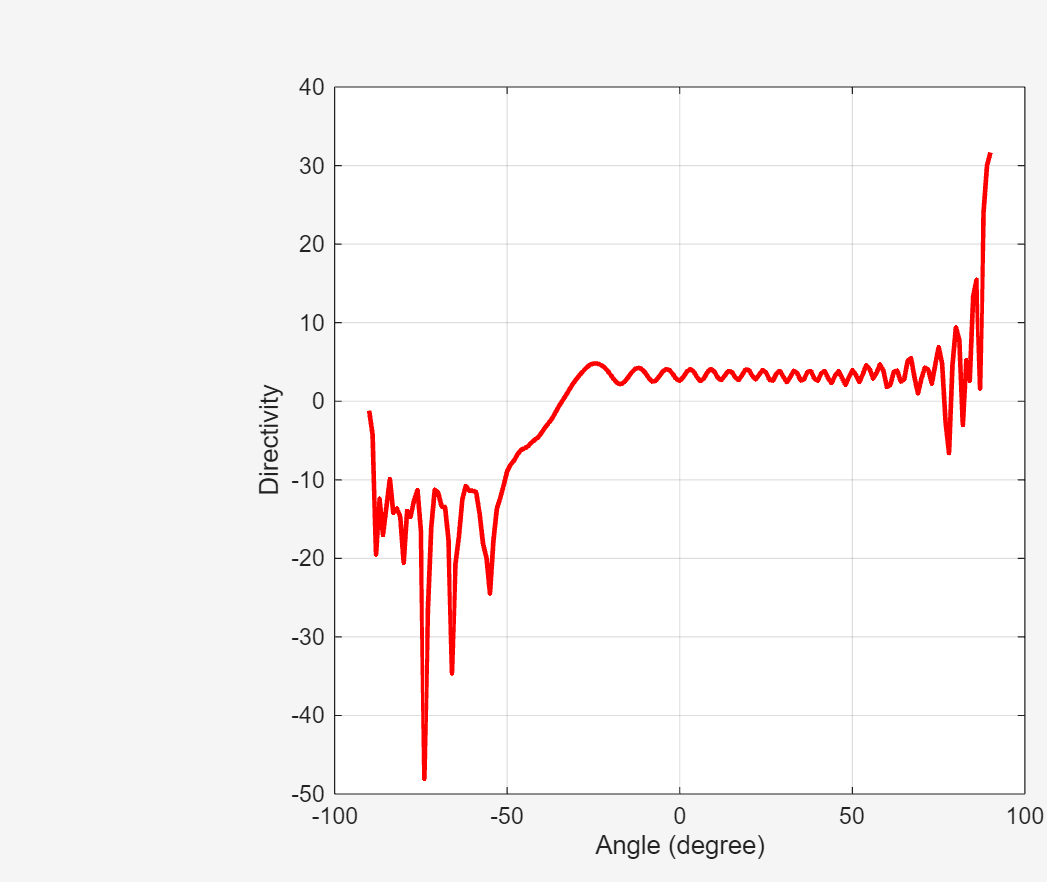The plot shows that although the maximum value for the directivity does not change by much, the nulls in the pattern are resolved more accurately. Note the approximately 20 dB drop in minimum value of directivity registered.

Plot the convergence of the solver. Notice that, the relative residual is achieved this time in fewer iterations, but the computation takes more time, since mesh refinement results in a greater number of unknowns to solve.

```figure convergence(s)```